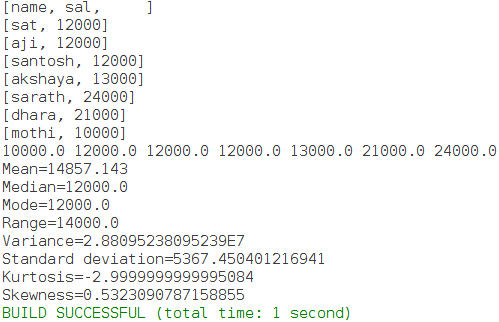• #5, First Floor, 4th Street Dr. Subbarayan Nagar Kodambakkam, Chennai-600 024 Landmark : Samiyar Madam
• pro@slogix.in
• +91- 81240 01111

### How to calculate Skewness and Kurtosis from a file in java?

#### Description

To Find the value of skewness and kurtosis from a file in java.

###### Process
Skewness:

It is a measure of the asymmetry of the probability distribution of a real-valued random variable about its mean.

The skewness value can be positive or negative, or undefined.

Kurtosis:

Kurtosis is the measure of thickness or heaviness of the given distribution.

Its actually represents the height of the distribution.

###### Sample Code

package Stat;

import java.io.File;
import java.io.FileNotFoundException;
import java.io.IOException;
import java.io.PrintWriter;
import java.util.ArrayList;
import java.util.Arrays;
import java.util.Collections;
import java.util.Scanner;

public class mean {
public static void main(String[] args) throws FileNotFoundException, IOException {

PrintWriter out = new PrintWriter(new File("file2.csv"));
File file = new File("file2.csv");

int skipIndex = 1;
String line1;
while ((line1 = in.readLine()) != null) {
String[] parts = line1.split(" ");
System.out.println(Arrays.toString(parts));
StringBuilder outLine = new StringBuilder();
for (int i = 0; i < parts.length; i++) {
if (i + 1 != skipIndex) {
outLine.append(" " + parts[i]);
}

}

out.println(outLine.toString().trim());

}

in.close();
out.close();

String line;

Scanner sc = null;

try {
sc = new Scanner(file);
sc.next();
} catch (FileNotFoundException e) {
e.printStackTrace();
return;
}

ArrayList list = new ArrayList();

while (sc.hasNextFloat())

int size = list.size();

if (size == 0) {
System.out.println("Empty list");
return;
}

Collections.sort(list);

for (int i = 0; i < size; i++) {
System.out.print(list.get(i) + " ");
}

System.out.println();

// mean value
float sum = 0;
for (float x : list) {
sum += x;
}

float mean = sum / size;
#variance
double variance;
double m = mean;
double temp = 0;
for(double a :list)
temp += (a-m)*(a-m);
variance= temp/(size-1);
double sd=Math.sqrt(variance);
# Skewness
double skew1=mean-mode;
double skew=skew1/sd;
#Kurtosiss
double kurt1=skew1/size;
double kurt=(kurt1/sd1)-3;

System.out.println("Skewness=" + skew);
System.out.println("Kurtosis=" + kurt);

}
}

###### Screenshots